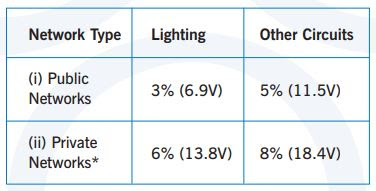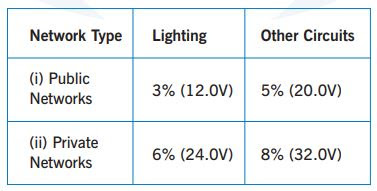Voltage drop in a consumer’s installation can be a contentious issue. However, it is an important aspect of installation design since if it is too high some certain equipment will not function correctly or will not function at all.

Rules Applied:

• 525.1 In the absence of any other consideration, under normal service conditions the voltage at the terminals of any fixed current-using equipment shall be greater than the lower limit corresponding in the product standard relevant to the equipment.
• 525.100 Where fixed current-using equipment is not the subject of a product standard the voltage at the terminals shall be such as not to impair the safe functioning of that equipment.
• 525.101 The above requirements are deemed to be satisfied if the voltage drop between the origin of the installation (usually the supply terminals) and a socket-outlet or the terminals of fixed current-using equipment, does not exceed that stated in Appendix 4 Section 6.4

#### Calculating Voltage Drop

When calculating voltage drop due consideration should be given to the following:
• motor starting currents; in-rush currents
• control voltages (particularly those associated with computerized systems).

Notes:
1. Motor control contactors and relays can ‘drop out’ if the coil voltages fall towards 80% of the operating voltage.
2. The effects of harmonic currents may also need to be considered and included in the calculation.
3. Voltage transients and voltage variations due to an abnormal operation can be ignored.

The maximum voltage drop values can be taken from the table shown below:

For 230 Volts NetworkAllowable Voltage Drop Based on BS 7671

*The voltage drop within each final circuit on Private Networks, should not exceed the values given in (i) above for Public Networks

For 400 Volts NetworkAllowable Voltage Drop Based on BS 7671

*The voltage drop within each final circuit on Private Networks, should not exceed the values given in (i) above for Public Networks

When calculating the voltage drop in a circuit, the design current can be taken as being either the equipment rated current or, where there are a number of loads, the total connected load multiplied by a diversity factor.

Note: If the total circuit length exceeds 100 meters, the limits given in Table 4Ab may be increased by 0.005% per meter up to a maximum of 0.5%.

The voltage drop can be apportioned throughout the system circuits as the designer wishes, but the final circuit voltage drop is limited to the values given for Public Networks, regardless of whether it is a Public Network or a Private Network.

In case that the supply voltage at the origin is lower than the nominal 230/400V, the designer needs to consider the effect of the minimum permissible supply voltage. This is a maximum of 6% below the nominal supply voltage, which equates to 216.2V (for 230 V network ) and 376V (for 400 V network) respectively.

Reference:

1.2.3.